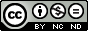### Soliton solutions of nonlinear fractional differential equations with its applications in mathematical physics

A. C. Çevikel, E. Aksoy

#### Abstract

Generalized Kudryashov method has been used to private type of nonlinear fractional differential equations. Firstly, we proposed a fractional complex transform to convert fractional differential equations into ordinary differential equations. Three applications were given to demonstrate the effectiveness of the present technique. As a result, abundant types of exact analytical solutions are obtained.

#### Keywords

Exact solutions, Modified Riemann-Liouville derivative, Fractional complex transform, fractional differential equations

PDF

#### References

Hilfer R., Applications of Fractional Calculus in Physics, World Scientific, River Edge, New Jersey, 2000.

Kilbas A., Srivastava H. M., Trujillo, J. J., Theory and Applications of Fractional Differential Equations, Elsevier, Amsterdam (2006)

Miller K. S., Ross B., An Introduction to the Fractional Calculus and Fractional Differential Equations, Wiley, New York (1993)

Podlubny I., Fractional Differential Equations, Academic Press, California (1999)

Sabatier J., Agrawal O.P., Machado J.A.T., (Eds.), Advances in Fractional Calculus: Theoretical Developments and Applications in Physics and Engineering, Springer, Dordrecht, 2007.

Bekir A., Güner Ö., Cevikel A.C., Fractional Complex Transform and exp-Function Methods for Fractional Differential Equations, Abstract and Applied Analysis, 2013, (2013) 426462

Bekir A., Güner Ö., Cevikel A.C., A Procedure to Construct Exact Solutions of Nonlinear Fractional Differential Equations, The Scientific World Journal, 2014, (2014) 489495.

Cevikel A.C., New Exact Solutions of The Space-Time Fractional KdV-Burgers and Non-Linear Fractional Foam Drainage Equation, Thermal Science, 22, (2018) 15-24.

Bekir A., Güner Ö., Cevikel A.C.,The Exp-function Method for Some Time-fractional Differential Equations, IEEE-CAA Jornal of Automatica Sinica, 4 (2017) 315-321.

Bekir A., Güner Ö., Ayhan B., Cevikel A.C., Exact Solutions for Fractional Differential-Difference Equations by (G '/G)-Expansion Method with Modified Riemann-Liouville Derivative, Advances in Applied Mathematics and Mechanics, 8, 2 (2016) 293-305.

Guner O., Aksoy E., Bekir A., Cevikel A.C., Different methods for (3+1)-dimensional space-time fractional modified KdV-Zakharov-Kuznetsov equation, Computers & Mathematics with Applications, 71 (2016) 1259-1269.

Shang N., Zheng B., Exact Solutions for Three Fractional Partial Differential Equations by the (G′/G) Method, International Journal of Applied Mathematics, 43 (2013) 3

Guner O., Bekir A., Cevikel A.C., A variety of exact solutions for the time fractional Cahn-Allen equation, European Physical Journal Plus, 130, 7 (2015).

Eslami M., Vajargah B. F., Mirzazadeh, M., Biswas, A., Application of first integral method to fractional partial differential equations, Indian J. Phys. 88, 2 (2014) 177--184

Aksoy E., Cevikel A.C., Bekir A., Soliton solutions of (2+1)-dimensional time-fractional Zoomeron equation, Optik, 127,17, (2016) 6933-6942.

Zheng B., Wen C., Zheng, B., Wen, C., Exact solutions for fractional partial differential equations by a new fractional sub-equation method, Advances in Difference Equations, 2013 (2013) 199

Alzaidy J. F., Fractional Sub-Equation Method and its Applications to the Space-Time Fractional Differential Equations in Mathematical Physics, British Journal of Mathematics & Computer Science, 3 (2013) 153-163

Bekir A., Aksoy E.,Cevikel A.C., Exact solutions of nonlinear time fractional partial differential equations by sub-equation method, Mathematical Methods in The Applied Sciences, 38, 13 (2015) 2779-2784.

Aksoy E., Bekir A., Cevikel A.C., Study on Fractional Differential Equations with Modified Riemann-Liouville Derivative via Kudryashov Method, International Journal of Nonlinear Sciences and Numerical Simulation, 20, 5 (2019) 511-516.

Zerarka A., Ouamane S., Attaf A., On the functional variable method for finding exact solutions to a class of wave equations, Applied Mathematics and Computation, 217, 7 (2010) 2897-2904.

Taghizadeh N., Mirzazadeh M., Rahimian M., Akbari M., Application of the simplest equation method to some time-fractional partial differential equations, Ain Shams Engineering Journal, 4, 4 (2013) 897-902

Esen A., Yagmurlu N., M., Tasbozan O., Approximate Analytical Solution to Time-Fractional Damped Burger and Cahn-Allen Equations, Appl. Math. Inf. Sci., 7 5 (2013) 1951-1956

Jumarie G., Modified Riemann-Liouville derivative and fractional Taylor series of nondifferentiable functions further results, Comput. Math. Appl., 51 (2006) 1367--1376

Jumarie G., Table of some basic fractional calculus formulae derived from a modified Riemann-Liouvillie derivative for nondifferentiable functions, Appl. Maths. Lett., 22 (2009) 378-385

Bulut H., Kilinç B., Exact solutions for some fractional nonlinear partial differential equations via Kudryashov mothod, New World Sciences Academy, 8, 1 (2013) 24-31

Li Z.B., He J H., Fractional complex transform for fractional differential equations, Math. Comput. Appl., 15 (2010) 970-973

Li Z.B., He J. H., Application of the fractional complex transform to fractional differential equations Nonlinear Sci. Lett. A Math. Phys. Mech., 2 (2011) 121-126

Kudryashov N.A., One method for finding exact solutions of nonlinear differential equations, Commun Nonlinear Sci Numer Simulat 17 (2012) 2248-2253

DOI: https://doi.org/10.31349/RevMexFis.67.422

### Refbacks

• There are currently no refbacks.